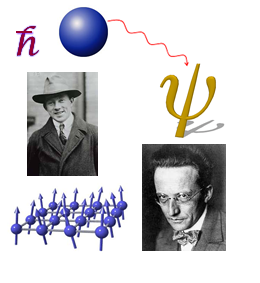# Epistemic Probability: Definition, Example

Share on

Key Takeaways:

• Epistemic probability is incomplete information about how probabilities arise.
• The field bridges the gap between known measurements and what is thought to be true.
• An example is classical statistical mechanics.

Epistemic probability concerns “…our possession of knowledge, or information.” It is incomplete knowledge about the mechanism from which the probabilities are derived. A common use of the term is to define how much support is given by all the available evidence; it can be measured in “degrees of belief” or “degrees of rational belief”.

The term Epistemic comes from philosophy and means:

“…namely the degree of belief in the occurrence of the state of affairs, the willingness to act on its assumption, a degree of support or confirmation, or similar” .

## Types of Epistemic Probability

Epistemic probability merges traditional statistics, approaching the problem from the viewpoint of epistemic attitudes. These probabilities can be:

• Doxastic: Opinions about data and hypotheses, with a strength of belief.
• Decision-theoretic: Builds on doxastic in a more elaborate way, figuring out dispositions towards actions and decisions relating to data and hypotheses.
• Logical: A formal framework for epistemic probability, comparable to truth values in deductive logic.

## Example of Epistemic Probability from Quantum MechanicsOne example of the use of epistemic probability is to assign probabilities to the possible truth of a proposed law of physics. In quantum mechanics, there are two different approaches to determining probabilities in quantum mechanics. The more fundamental way is through Brownian motion, a type of stochastic process. However, classical statistical mechanics, which is deterministic, is epistemic probability—the probabilities emerge because of incomplete descriptions . The gap between what is measured and what is thought to be true is achieved through development of an epistemic probabilistic framework.

References:
Image: Voyajer at the English-language Wikipedia, CC BY-SA 3.0, via Wikimedia Commons
 Stanford Encyclopedia of Philosophy. Philosophy of Statistics. Retrieved October 22, 2021 from: https://plato.stanford.edu/entries/statistics/
 Saunders, S. What is Probability? Retrieved October 22, 2021 from: https://citeseerx.ist.psu.edu/viewdoc/download?doi=10.1.1.252.8105&rep=rep1&type=pdf

CITE THIS AS:
Stephanie Glen. "Epistemic Probability: Definition, Example" From StatisticsHowTo.com: Elementary Statistics for the rest of us! https://www.statisticshowto.com/epistemic-probability/
---------------------------------------------------------------------------Need help with a homework or test question? With Chegg Study, you can get step-by-step solutions to your questions from an expert in the field. Your first 30 minutes with a Chegg tutor is free!